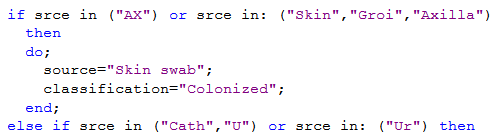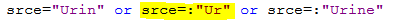## Efficient way for if, else if, then do statements

Hi,

I have the following dataset sample and code which has a lot of if, if else, then do statements. I just wanted to check if there is a better way to write this code. Any help is really appreciated.

``````data Test;
infile datalines dlm=',' dsd missover;
Length notes \$145 srce \$145;
input hosp \$ org \$ srce \$ notes \$;
datalines;
ABC,Strep,AX,this is a test
ABC,Kleb,Groi,test
ABC,Kleb,Sput,
ABC,Auris,Other,SourceSputum
ABC,Auris,U,
ABC,Auris,Urine,
ABC,Ecoli,Blood,This is test
ABC,Kleb,Bld,
XYZ,Kleb,Groin,
XYZ,Ecoli,Other,Blood test
XYZ,Kleb,Ur,
XYZ,Ecoli,Wound,
XYZ,Kleb,Fluid,WD test
XYZ,Ecoli,Other,BldTest
XYZ,Auris,Body Fluid,
XYZ,Auris,Resp,
RTY,Kleb,,Test
RTY,Ecoli,,Other Resp
RTY,Kleb,Blood,BloodSrce
RTY,Kleb,Resp,
RTY,Ecoli,Cath,
RTY,Ecoli,Ur,
RTY,Auris,Wnd,Srce
RTY,Auris,Bd,Srcetest
RTY,Auris,Rectal,Srce
HOW,Kleb,Other,Fluid Test
HOW,Ecoli,Other,Blood source test in body
HOW,Kleb,wound,
HOW,Kleb,Fluid Specimen,RespTesing in body
HOW,Ecoli,Other,None
HOW,Ecoli,Cath,
HOW,Auris,Blood,
HOW,Auris,Venous,
HOW,Auris,Ven,
CVS,Kleb,Skin,
CVS,Ecoli,Axilla,
CVS,Kleb,Other,
CVS,Kleb,Gr,
CVS,Ecoli,Vein,
CVS,Ecoli,Aspirate,
CVS,Auris,Trach,
CVS,Auris,Blood,Test
CVS,Auris,Urin,UrineSource
;;;
run;
data source;
set test;
length source \$145 classification \$145;
notes=upcase(notes);

if srce="AX"
or srce=:"Skin"
or srce=:"Groi"
or srce=:"Groin"
or srce=:"Axilla"
or srce="Gr"
then do;
source="Skin swab";
classification="Colonized";
end;

else if srce="Cath"
or srce="U"
or srce="Urin"
or srce=:"Ur"
or srce=:"Urine"
then do;
source="Urine";
classification="Clinical";
end;

else if srce=:"Bd"
or srce=:"vein"
or srce=:"Blood"
or srce=:"Bld"
or srce=:"ven"
or srce="Venous"
then do;
source="Blood";
classification="Clinical";
end;

else if srce="Resp"
or srce="sputum"
or srce="Throat"
or srce="Aspirate"
or srce=:"Trach"
or srce="Sput"
then do;
source="Respiratory";
classification="Clinical";
end;

else if srce="Wound"
or srce="Wnd"
then do;
source="Wound";
classification="Clinical";
end;

else if srce="Fluid"
or srce="Body flu"
or srce="Rectum"
or srce="Fluid Test"
or srce=:"rectal"
then source="Unknown";

else if srce="Other"
then do;
source="Other";
classification="Clinical";
end;
else source=srce;

if (srce="" or srce="Other")
and (index(notes, 'BLOOD')>0) and ((index(hosp, 'XYZ')>0) or (index(hosp, 'HOW')>0))
then do;
source="Blood";
classification="Clinical";
end;
else if (srce=" " or srce="Other")
and (index(notes, 'RESP')>0)
then do;
source="Respiratory";
classification="Clinical";
end;
else if (srce="" or srce="Other")
and (index(notes, 'URINE')>0)
then do;
source="Urine";
classification="Clinical";
end;
else if (srce="" or srce="Other")
and (index(notes,'SPUTUM')>0)
then do;
source="Respiratory";
classification="Clinical";
end;
else if (srce="" or srce="Other")
and (index(notes, 'FLUID')>0
or index(notes, ' ')>0)
then source="Other";
run;``````
1 ACCEPTED SOLUTION

Accepted Solutions

## Re: Efficient way for if, else if, then do statements

I can't see much that you could change except for using the IN operator. Below how your first two conditions could look like:You can also remove some of your tests because they are already contained in other tests. Like below the highlighted test already covers the other two tests.4 REPLIES 4

## Re: Efficient way for if, else if, then do statements

I can't see much that you could change except for using the IN operator. Below how your first two conditions could look like:You can also remove some of your tests because they are already contained in other tests. Like below the highlighted test already covers the other two tests.## Re: Efficient way for if, else if, then do statements

Thank you, Patrick, this really helps.

## Re: Efficient way for if, else if, then do statements

Just some small considerations...  Some of your comparisons are redundant and can be removed.  For example, consider:

``````            or srce=:"Groi"
or srce=:"Groin"``````

The first comparison will automatically select any values that the second condition would pick up.  The second comparison can be removed.  SImilarly here:

``````            or srce="Urin"
or srce=:"Ur"
or srce=:"Urine"``````

The middle comparison is all that is needed, since it selects all cases that meet the other two conditions.  Reduce the three to:

``````            or srce=:"Ur"
``````

## Re: Efficient way for if, else if, then do statements

Thank you astounding.
Discussion stats
• 4 replies
• 159 views
• 0 likes
• 3 in conversation